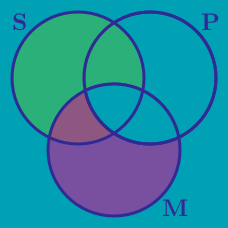Logic

# Intermediate Syllogistic Logic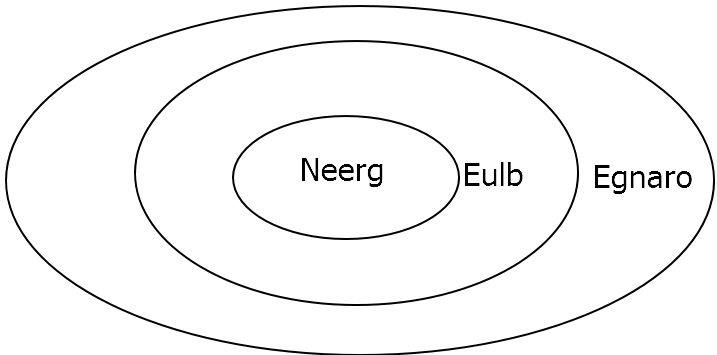True or False?

Based on the diagram above, all Neergs are Eulbs, but some Egnaros are Eulbs. (You can assume each area on the diagram contains at least one thing.)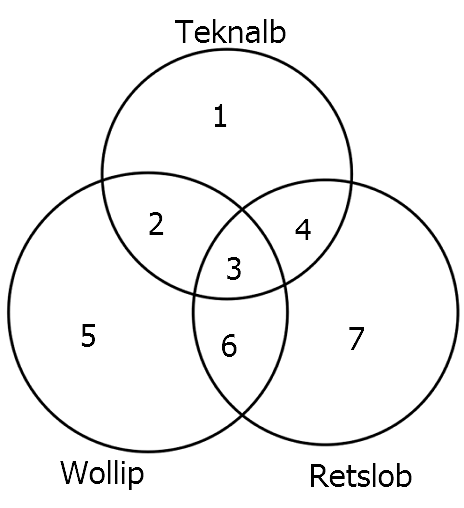In the Venn diagram above, the number inside each of the 7 regions represents the number of people who fall into that category. For example, 1 person is a Teknalb but neither a Wollip nor a Retslob.

How many people are Wollip but not Retslob?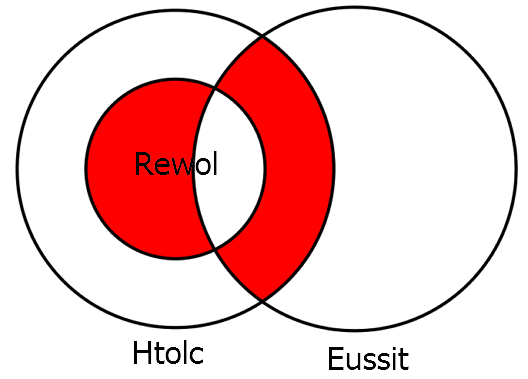Which of the following statements is not true about the Venn diagram above?

All Lannisters pay debts.

Which of the following diagrams are consistent with the above statements? (You can assume each portion of the diagram is filled by at least one element.)

A.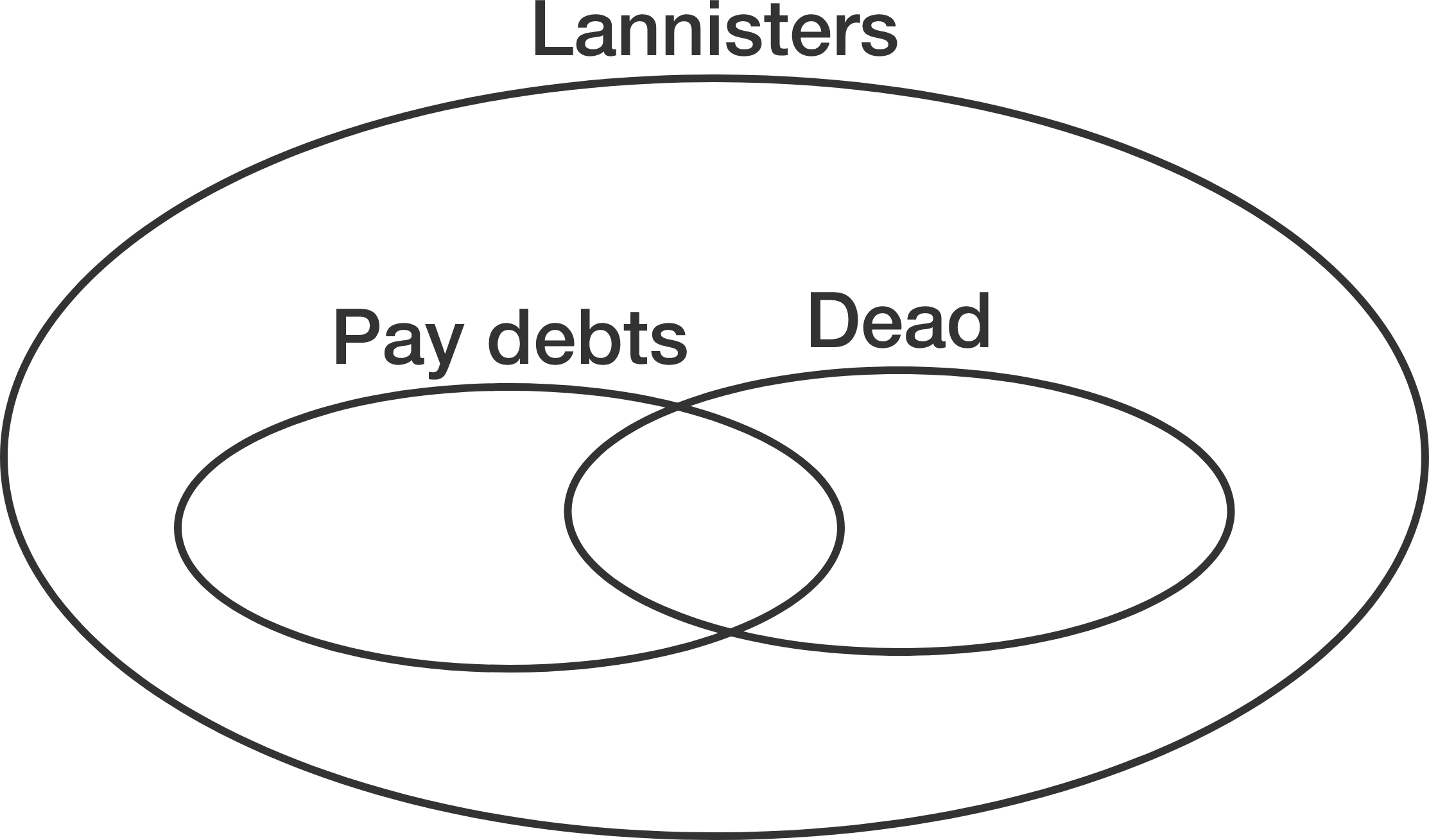B.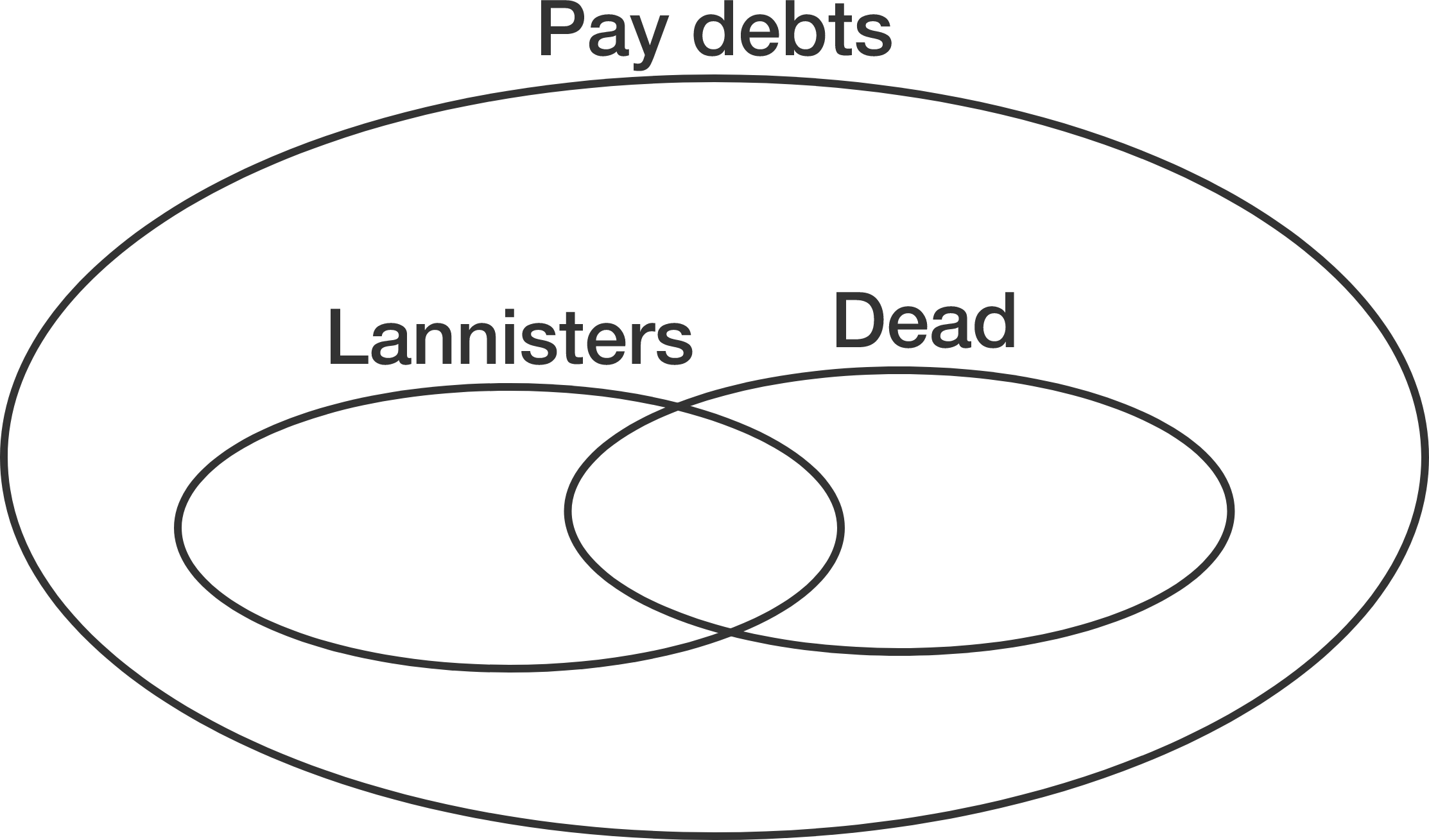C.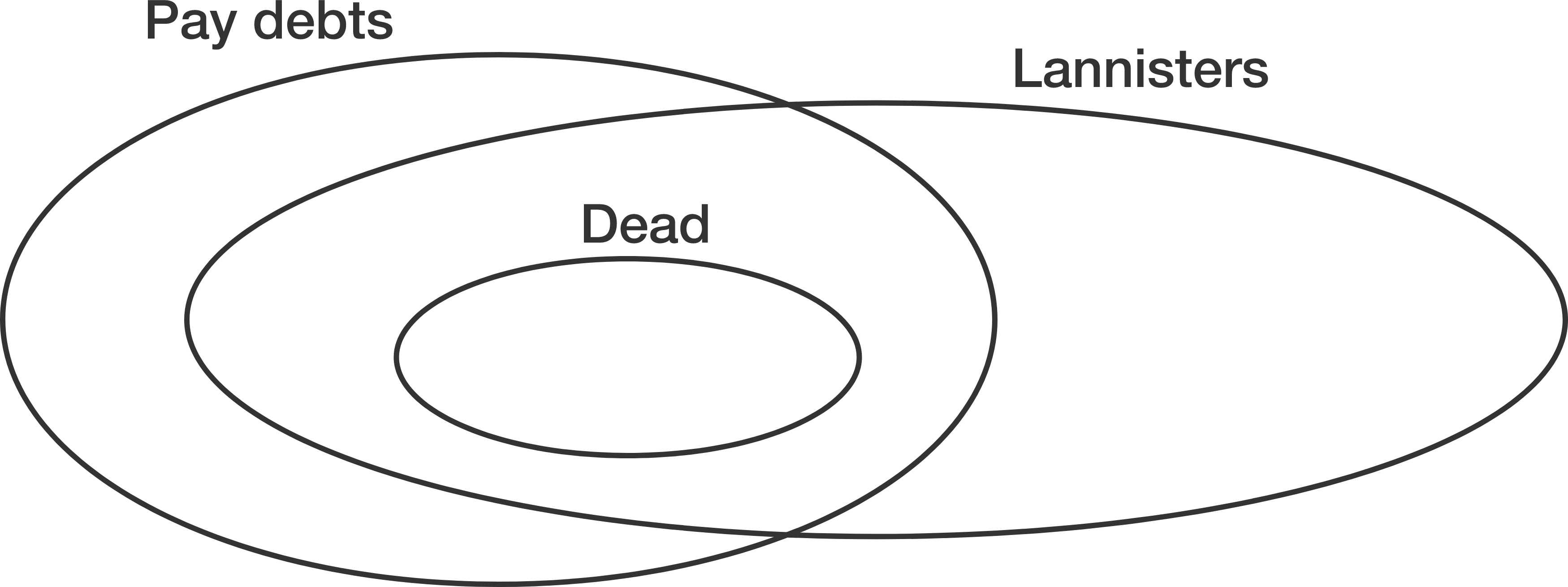D.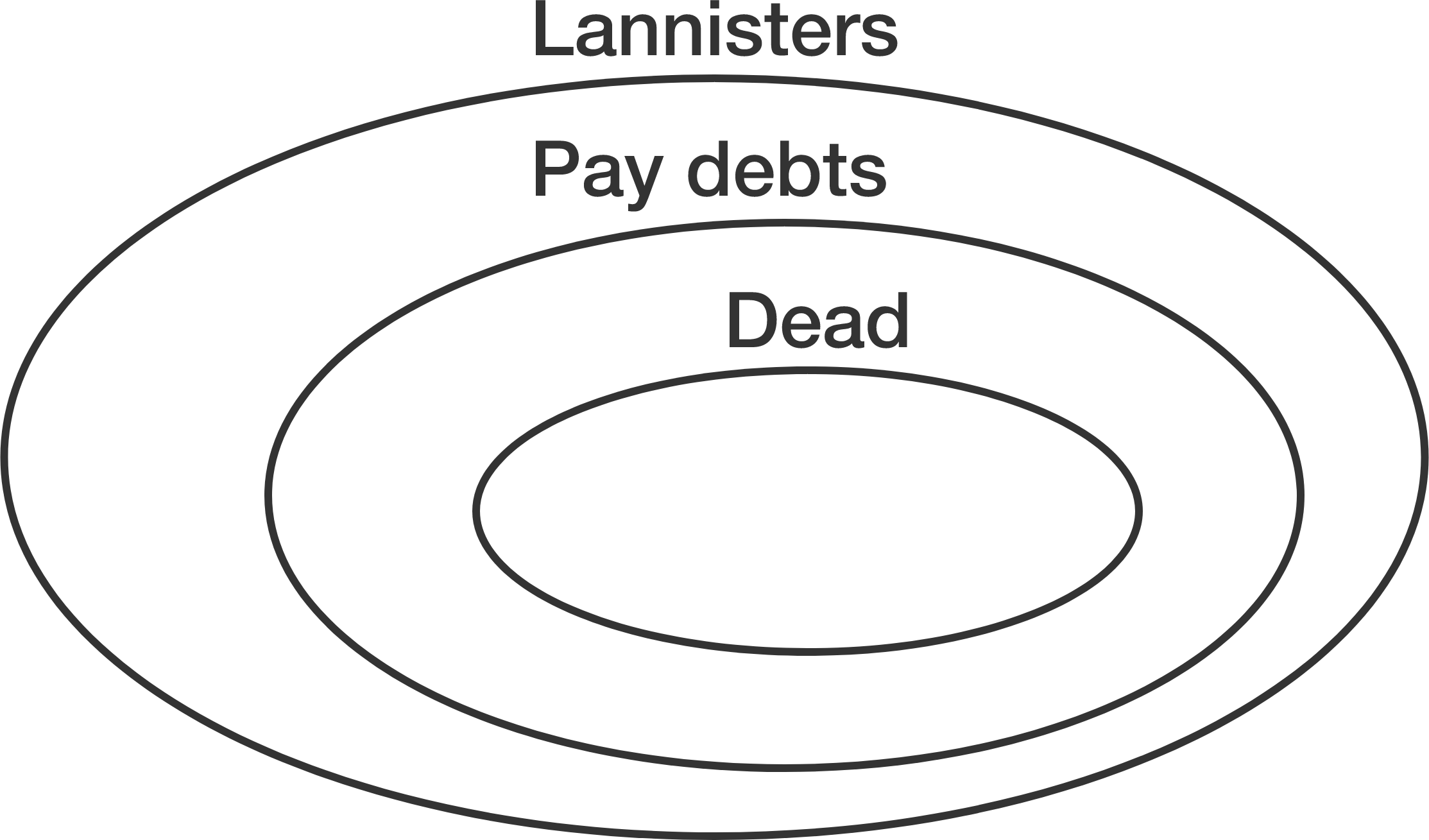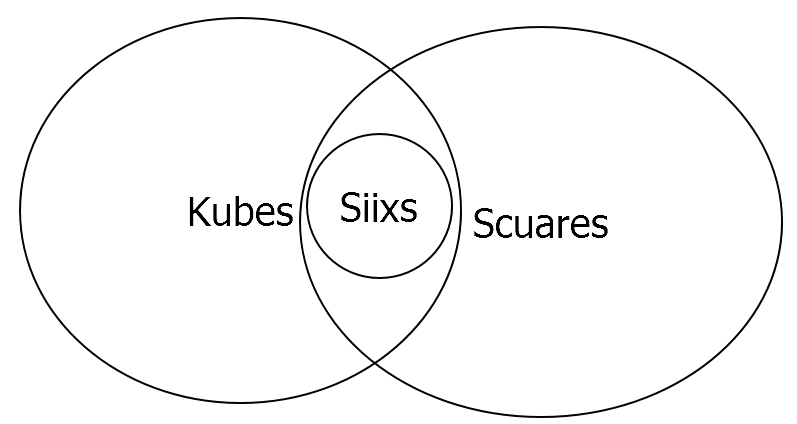True or False?

Based on the diagram above, all Siixs are Kubes, but not all Siixs are Scuares.

×